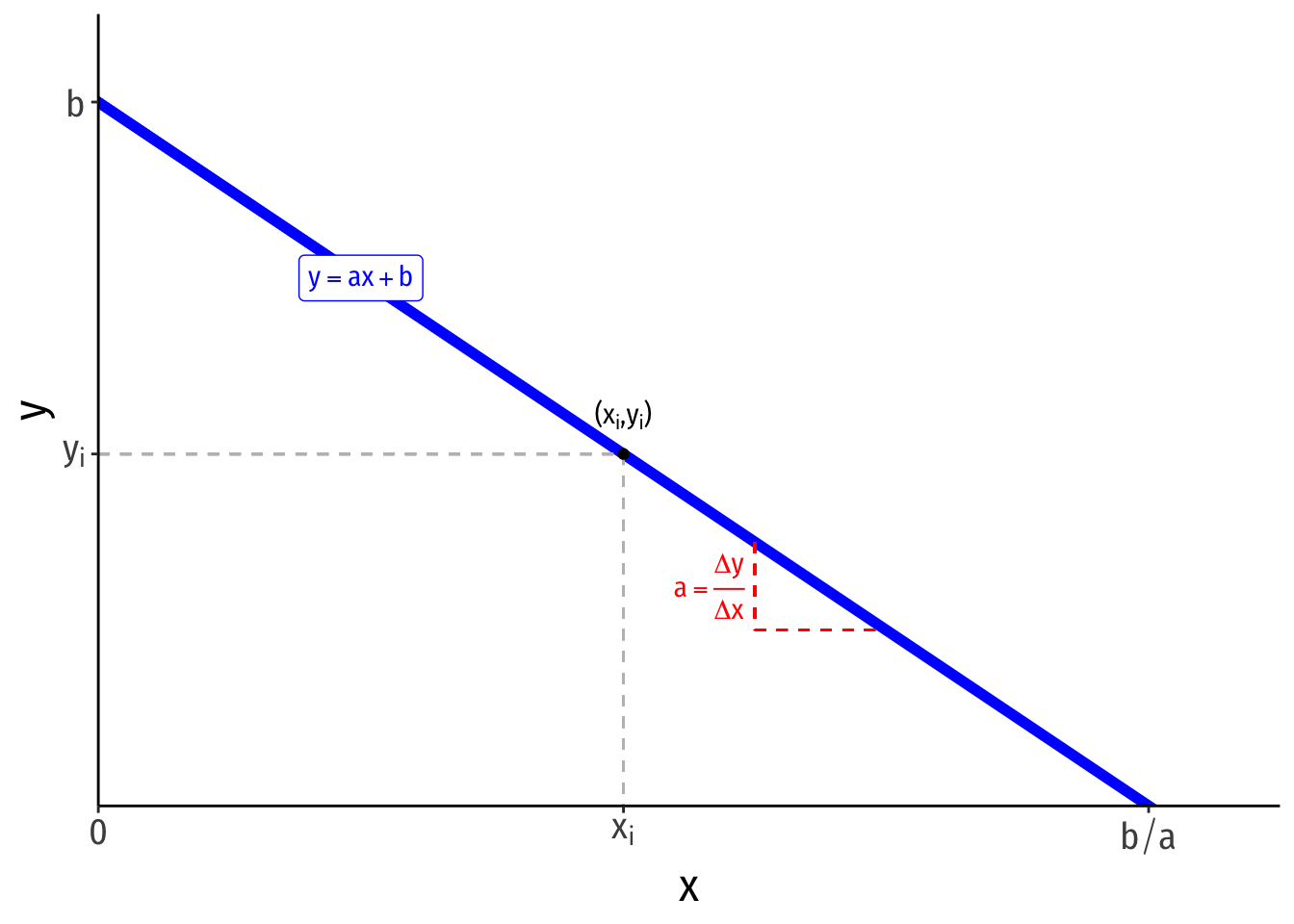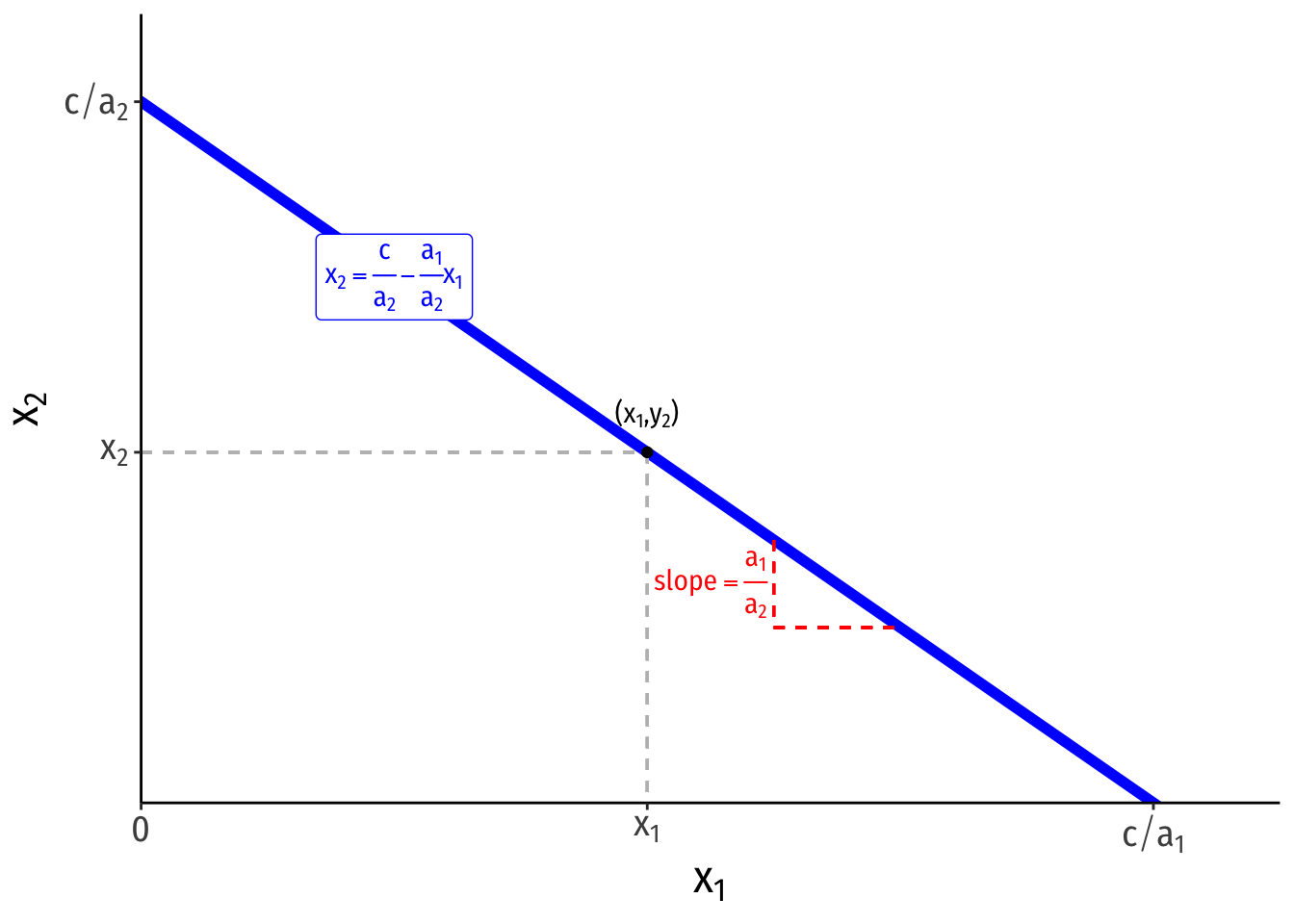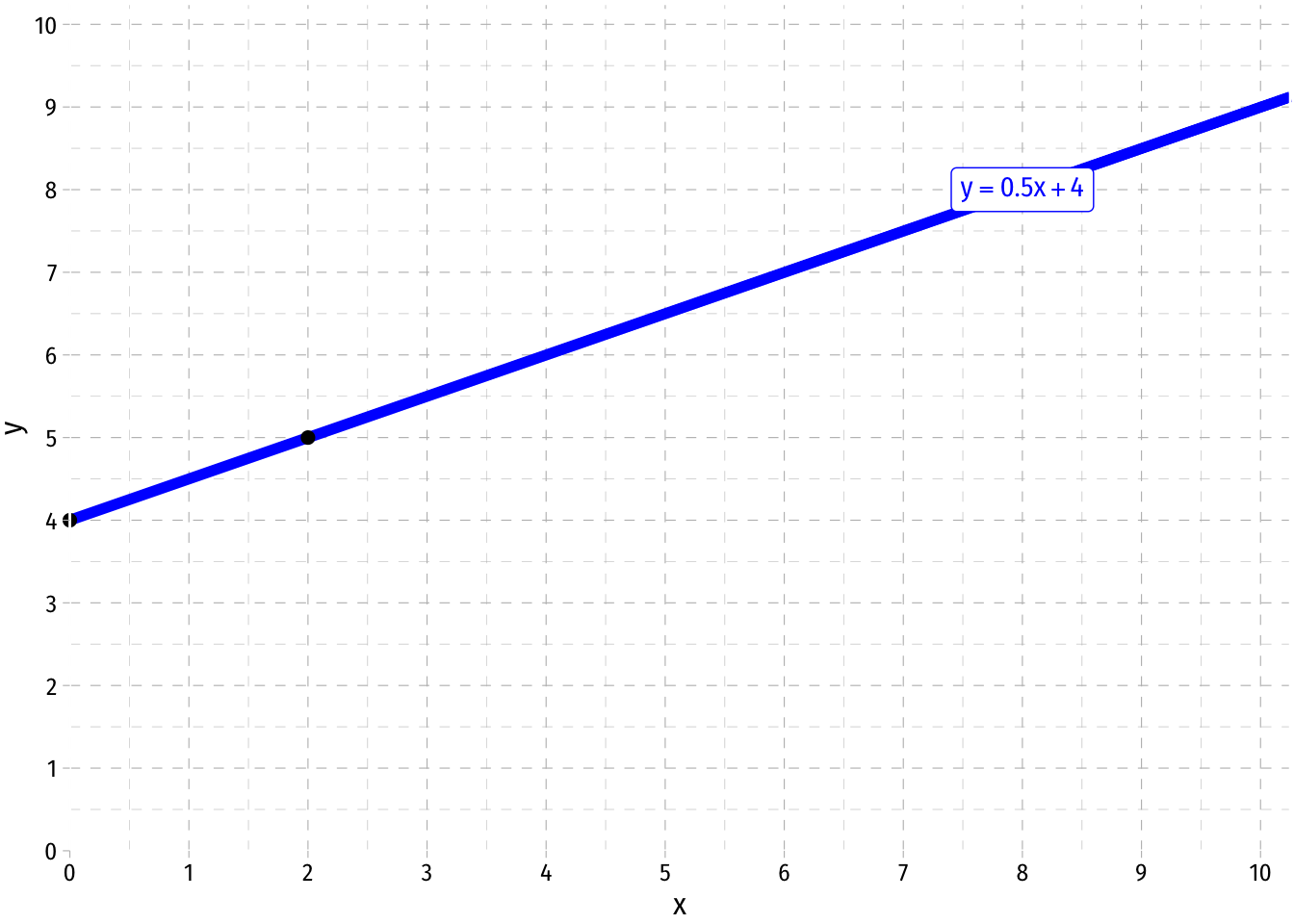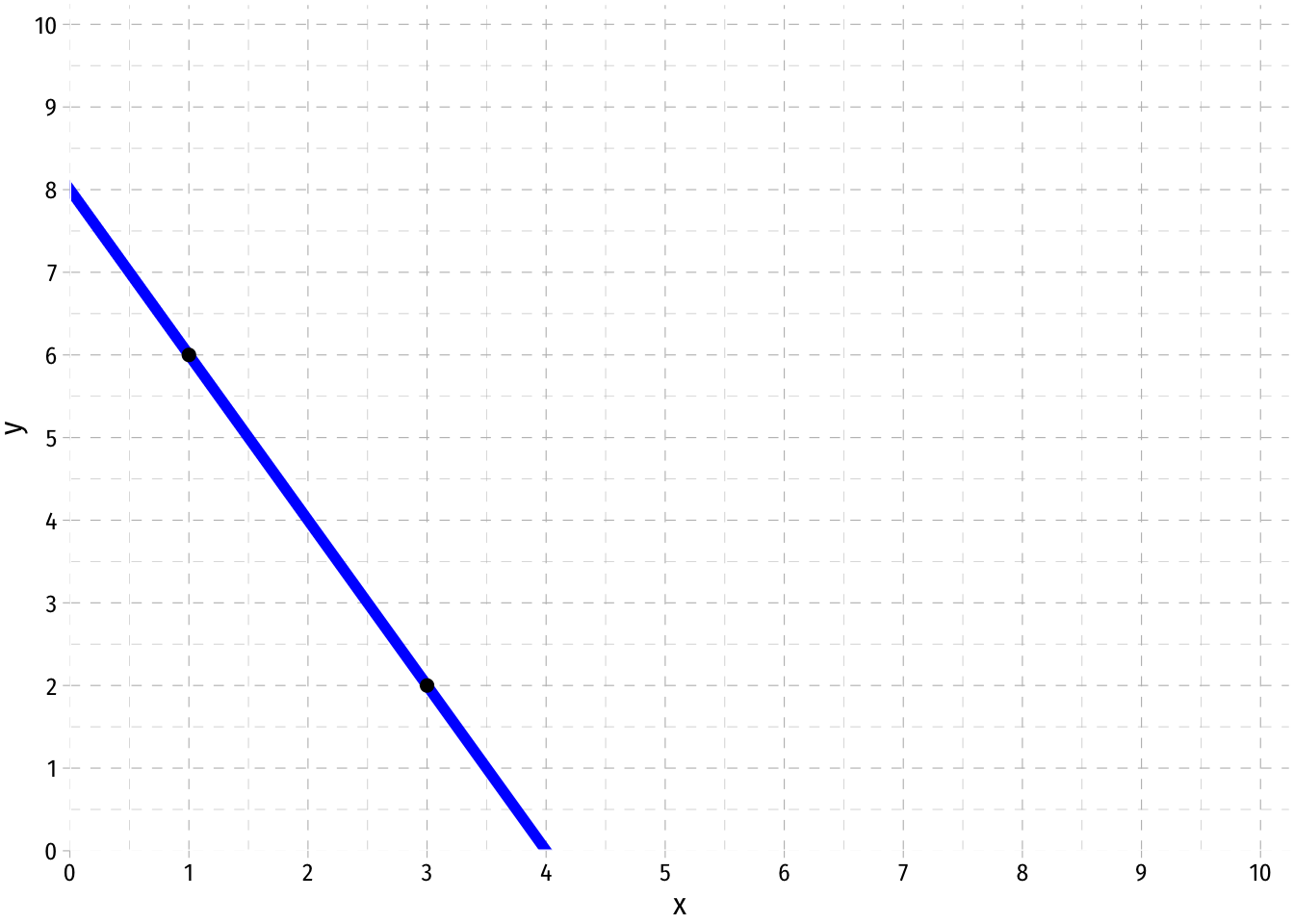## Slope-Intercept Form• A linear function of two variables can be written in slope-intercept form:

$y=ax+b$

• $$y$$ is the dependent variable (on the vertical axis)
• $$x$$ is the independent variable (on the horizontal axis)
• $$a$$ is the slope of the line
• $$a = \frac{\text{rise}}{\text{run}} = \frac{\text{change in }y}{\text{change in }x} = \frac{\Delta x}{\Delta y}$$
• you may have been taught the slope as “$$m$$”, this is just personal taste, but again, get used to using different letters!
• $$b$$ is the vertical-intercept, a constant number where the line crosses the vertical axis
• if $$y$$ is the dependent variable, this is the “$$y$$-intercept”, $$y$$ where $$x=0$$
• Any point on the line has an $$x$$-coordinate and $$y$$-coordinate $$(x_i,y_i)$$

## Other Forms

• A linear function can equivalently be expressed in the following form:

$a_1x_1+a_2x_2=c$

• $$x_2$$ is the dependent variable (on the vertical axis)

• $$x_1$$ is the independent variable (on the horizontal axis)

• $$c$$ is a constant

• This is a valid equation, but is difficult to visualize in the traditional graph as above. Simply solve for the dependent variable on the vertical axis $$(x_2$$ as if you were solving for $$y)$$:\begin{align*} a_1x_1+a_2x_2=c && \text{Original}\\ a_2x_2=c-a_1x_1 && \text{Subtracting }x_1 \text{ term}\\ x_2 = \frac{c}{a_2}-\frac{a_1}{a_2}x_1 && \text{Dividing by }a_2 \\ \end{align*}

• The vertical intercept is $$\frac{c}{a_2}$$

• The horizontal intercept is $$\frac{c}{a_1}$$

• The slope is $$-\frac{a_1}{a_2}$$

• This is extremely useful for dealing with constraints in constrained optimization problems: budget constraints and isocost lines

## Drawing a Graph From an Equation

If we already have a linear equation that we would like to graph, we can follow these steps:

1. Take the equation and plug in two values, e.g. if we have:

$p = \frac{1}{2}q + 4$

1. We can find two points on the graph. The easiest one to find is the vertical-intercept, where the line crosses the vertical axis, where $$q=0$$, so plug in $$q=0$$:

\begin{align*} p &= \frac{1}{2}(0)+4\\ p &= 4 \\ \end{align*}

Thus, one point is $$(0,4)$$. Note that the constant in the function itself is the $$p-intercept$$! So one valid point will always be $$(0,b)$$!

1. For our second point, let’s plug in $$q=2$$:

\begin{align*} p &= \frac{1}{2}(2)+4\\ p &= 5 \\ \end{align*}

Thus, another point is $$(2,5)$$

1. Now, plot the two points on the graph, and connect them with a lineNote: A quick shortcut to plot a line is to find the vertical intercept and plot that, and then find the next point using the slope. Here, start our line at 4 on the vertical axis, and then, as the slope is $$\frac{1}{2}$$, for every one unit increase in $$q$$, $$p$$ increases by $$\frac{1}{2}$$. Our second point, (2,5), is a 2 unit increase in $$q$$ resulting in a $$1$$ unit increase in $$p$$.

## Finding an Equation from a GraphIn order to find the equation of an existing line, we follow these steps:

1. First, take two points on the line and find the slope, $$a$$, between them. Let’s pick $$(1,6)$$ and $$(3,2)$$.

\begin{align*} \text{Slope} &= \frac{rise}{run} \\ &= \frac{(p_2-p_1)}{(q_2-q_1)}\\ &= \frac{(2-6)}{(3-1)}\\ &= \frac{-4}{2}\\ &= -2\\ \end{align*}

There is a shortcut that we can use to find the slope faster by eye-balling the graph: When $$q$$ changes by 1, how many units does $$p$$ change? If we move from (1,6) to (2,5), $$q$$ increases by 1, but $$p$$ falls by 2. So the slope is $$-2$$. For every one unit increase in $$q$$, $$p$$ changes by -2.

1. Now with the slope, we need to find the vertical intercept, or $$b$$, we solve this by plugging in the slope and any point on the graph, we will use (1,6):

\begin{align*} p &= aq+b\\ (6) &= -2(1)+b\\ 6 &= -2+b\\ 8 &= b\\ \end{align*}

Note, there is another easy way to eye-ball what this value is. It is simply that $$p$$ value where $$q=0$$, or at what $$p$$ value the graph crosses the vertical axis. We can see it is at 8.

1. Thus, we have the slope and the intercept, so our equation is:

$p = -2q+8$